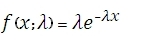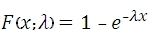# SQL Server EXPON.DIST function

Updated: 9 August 2010

Use EXPONDIST to calculate an exponential distribution that is equivalent to the function used in EXCEL. Exponential distributions describe times between events in a Poisson process, i.e. a process in which events occur continuously and independently at a constant average rate.

The equation for the probability density function is:The equation for the cumulative distribution function is:Syntax
SELECT [wctStatistics].[wct].[EXPONDIST] (
<@X, float,>
,<@Lambda, float,>
,<@Cumulative, bit,>)
Arguments
@X
is the value of the function. @X is an expression of type float or of a type that can be implicitly converted to float
@Lambda
is the parameter value. @Lambda is an expression of type float or of a type that can be implicitly converted to float
@Cumulative
is a logical value that determines the probability density function (False, 0) or the cumulative distribution function (True, 1) is being calculated.
Return Types
float
Remarks
·         If @X < 0, EXPONDIST returns an error.
·         If @Lambda ≤ 0, EXPONDIST returns an error
·         EXPONDIST = WEIBULL(@X,1,1/@Lambda, @Cumulative)
Examples

select
wct.EXPONDIST(0.5, 9.5, 'False')

This produces the following result

----------------------
0.082191104429646

(1 row(s) affected)

select

This produces the following result

----------------------
0.0821911044296461

select
wct.EXPONDIST(0.5, 9.5, 'True')

This produces the following result

----------------------
0.991348304796879

(1 row(s) affected)

select

This produces the following result

----------------------
0.991348304796879

(1 row(s) affected)

### SupportCopyright 2008-2023 Westclintech LLC         Privacy Policy        Terms of Service